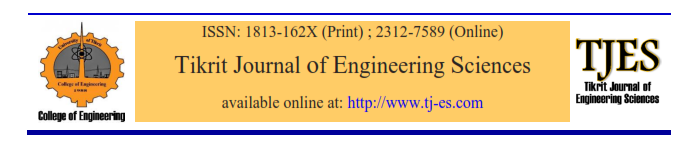# vol16no2pa8

References

 A.J.Willmott,,” Digital Computer Simulation Of a Thermal Regenerator”, .(Int.J.Heat Mass Transfer) .Vol .7,pp. 1291-1302 ,( 1964) .

 W.M.Kays&A.L.London.,”Compact Heat Exchanger”, 2th Edition Mcgraw Hill Book Company. NEWYORK .(1964).

AL-WanDhh “Modeling Of Rotating Regenerative Heat Pack “M.SC .Thesis , Baghdad University (1995).

 AL-Doury “The Qualitative and Quantitative Enhancement of Baiji Power Station Combustion Air” MSc Thesis, Tikrit University (2001).

شامل محمد الطالب “دراسة انخفاض كفاءة مسخنات الهواء الدوارة في مرجل محطة بيجي البخارية” رسالة ماجستير ،جامعة الموصل (2002)

 6-Yunus .A.Gengel&Michaal A. Boles ” Thermodynamics An Engineering” Approach .3rd Editions (1998) , Mcgraw-Hill. Regenerator”, .(Int.J.Heat Mass Transfer) .Vol .7,pp. 1291-1302 ,( 1964) .

 حسن مجيد الدلفي ” التحليل الهندسي والعددي والتطبيقي” 1999.

 8-.Frank .P.Incropera and David P.Dewitt ” Introduction To Heat Transfer ,3rd Edition (1996) USA.

Tikrit Journal of Engineering Sciences (2009) 16(2) 51- 65Investigating the Matrix Geometry Shape Effects on the performance of the Rotary Heat Exchanger in the Dorah Power Plant

 Thamer K. Ibraheemi Mech. Eng. Dept., Tikrit University , Iraq

Abstract

The present study is a theoretical investigation for the effects of several types of metal matrix for the rotary heat exchanger. Numerical analysis is used to analyze the parameters of the original matrix and compare them with those of the new three matrix: A metal matrix with a wave of (Corrugated Flat), a wave of ( Notched Flat ) , and tube matrix . The studied parameters included: temperature distribution of the combustion air , exhaust gases; heat transfer, pressure drop, heat elements, and surface area. It was seen that rate temperature of the burned air for the original matrix was 157.1 oC which is lower than that of TM and CF matrix by 14 % and 7.9 % respectively; and higher than that of the NF matrix by 1.6%. The temperatures of the exhaust gases increase during the cooling period with the increase of length of the heat exchanger. The mean temperature of exhaust gases for the original matrix was 296.6 oC which is higher than that of the TM and CF matrix by 3.7 % and 1.81 respectively; and lower than of the TM by 3.33%. The TM matrix has a heat transfer and pressure drop higher than the others. This is because the heat transfer coefficients of CF and NF matrix are lower than that of TM matrix by 8.2 % and 10.1 % respectively. The pressure drops for CF and NF matrix are lower by 45% and 53% respectively. The heat elements of TM matrix is higher than CF and NF by 65.4% and 76% respectively. The surface area decreases with the increase of cell number for all the types. TM matrix has the highest surface area. It is larger than that of CF and NF by 17.6% and 25% respectively.